# Maximum average of subtree values in a given Binary Tree

## Introduction

### Problem statement

We are given a Binary tree. Our goal is to find the maximum average of subtree values in a given binary tree.

The above problem statement means we need to find the maximum value from all the average values of the subtrees in a given binary tree.

• A subtree is any node on the tree with all its descendant nodes.

• The average value of the subtree =

The sum of values of all the nodes in the subtree / Total number of nodes in the subtree.

Example:

Input1: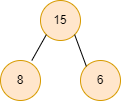Output1: 9.66667    //maximum average of subtree values

Explanation:

• Average value of subtree with node(15) = (15 + 8 + 6) / 3 = 9.66667  maximum average of subtree values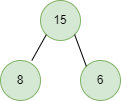• Average value of subtree with node(8) = 8 / 1 = 8.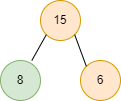• Average value of subtree with node(6) = 6 / 1 = 6.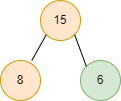Input2: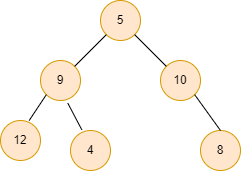Output2: 12     // maximum average of subtree values.

Explanation:

• Average value of subtree with node(5) = (5+9+12+4+10+8) / 6 = 8.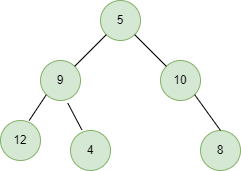• Average value of subtree with node(9) = (9+12+4) / 3 = 8.33333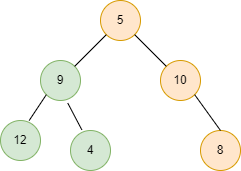• Average value of subtree with node(12) = 12 / 1 = 12.   //maximum average of subtree values.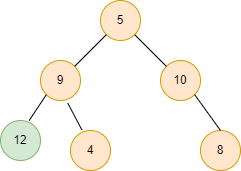• Average value of subtree with node(4) = 4 / 1 = 4.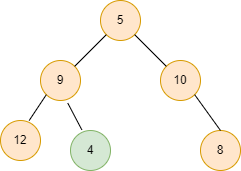• Average value of subtree with node(10) = (10+8) / 2 = 9.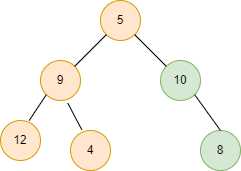• Average value of subtree with node(8) = 8 / 1 = 8.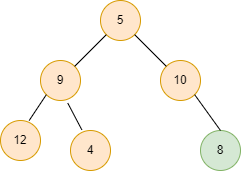## Approach

The idea is simple: we find the sum of all the nodes, count all the nodes for every subtree in the given binary tree, and calculate the average value (sum of all the nodes/count of nodes) for every subtree.

Sum of all the nodes in a subtree starting with a node = sum of all the nodes in the left subtree + sum of all the nodes in the right subtree + root node of the subtree.

Similarly, count of nodes in a subtree = count of nodes in the left subtree + count of nodes in the right subtree + 1 (for the root node of the subtree).

For calculating the count and sum of nodes, we will use the concept of recursion. Finally, we take the maximum of all the calculated average values and return the maximum average of subtree values.

Algorithm:

Declare a global maxm_avg = 0.0 variable of double data type to store the required maximum average of subtree values because the average value can be a decimal number.

In the main() function, call the maximum_avg_subtree() function, which calculates the maximum average value and passes the tree’s root node as an argument in it.

Steps for maximum_avg_subtree() function:

• Declare a count variable and initialise it with 0.
• Call subtree_sum function and pass root and count as an argument.
• Return the maxm_avg.

Steps for the subtree_sum() function:

• If root = NULL, the average value will be 0, so we return 0. It is the base case of our recursion.
• Create two variables, left_count = 0 and right_count = 0. It stores the count of the nodes in the left and right subtree, respectively.
• Create a left_sum variable that stores the sum of all the nodes in the left subtree, left_sum = subtree_sum(root->left, left_count).
• Similarly, create a right_sum variable that stores the sum of all the nodes in the right subtree, right_sum = subtree_sum(root->right, right_count).
• Calculate the sum of all the nodes of the subtree starting with any root, which is equal to the sum of all the nodes in the left subtree, right subtree and the value of the current root, sum = left_sum + right_sum + root->value.
• Similarly, calculate the count equal to the sum of the count of left subtree nodes, the count of right subtree nodes and 1 (for the current root node), count = left_count + right_count + 1.
• Now, calculate the maximum average seen so far, maxm_avg = max(maxm_avg, sum / count).
• Finally, return the sum.

Finally, print the maxm_avg, the maximum average of subtree values.

## Code in C++

``````#include<bits/stdc++.h>
using namespace std;

struct Node {
int val;
Node *left;
Node *right;
Node(int x)
{
val = x;
left = NULL;
right = NULL;
}
};

double maxm_avg = 0.0;

int subtree_sum(Node* root, int &count) {
if (root == NULL)
return 0;

int left_count = 0;
int right_count = 0;
int left_sum = subtree_sum(root->left, left_count);
int right_sum = subtree_sum(root->right, right_count);
int sum = left_sum + right_sum + root->val;
count = left_count + right_count + 1;
maxm_avg = max(maxm_avg, (double)sum / count);
return sum;
}
double maximum_avg_subtree(Node* root) {
int count = 0;
subtree_sum(root, count);
return maxm_avg;
}

int main()
{
Node* root = new Node(15);
root->left = new Node(8);
root->right = new Node (6);

cout << maximum_avg_subtree(root) << endl;

return 0;
}``````

Output

``9.66667``

### Complexity Analysis

Time complexity: O(n), where n is the number of nodes in the given tree as we are traversing all the nodes.

Space complexity: O(h), where h is the height of the given binary tree.

Q1. What is the purpose of a binary tree?

Ans: Binary trees are primarily used in computing for searching and sorting because they allow data to be stored hierarchically. Insertion, deletion, and traversal are some of the most common operations that can be performed on binary trees.

Q2. Is it possible for a binary tree to have only one child?

Ans: A binary tree is one in which no node has more than two children, and each child is either a left or right child, even if it is the parent's only child. A full binary tree is one with two children for each internal node.

Q3. What is the difference between a binary search tree and a binary tree?

Ans: A Binary Tree follows one simple rule: each parent node can have no more than two child nodes, whereas a Binary Search Tree is simply a variant of the binary tree that uses a relative order to organise the nodes in the tree.

Q4. How to recognise a leaf node in a binary tree?

Ans: Steps in C++:

• If root==NULL, return.
• If root->left==NULL and root->right==NULL, it is a leaf node, print the node data.
• Repeat the process for both the left and the right subtree.

## Key Takeaways

This article discussed the average value of a subtree and the problem, Maximum average of subtree values in a given Binary Tree with examples for better understanding and its C++ code.

If you are a beginner, interested in coding and want to learn DSA, you can look for our guided path for DSA, which is free!